# 11) Consider a large 5-cm-thick brass plate (k111 W/m-K) in whic uniformly at a rate of 200,000W Im3. One side of th...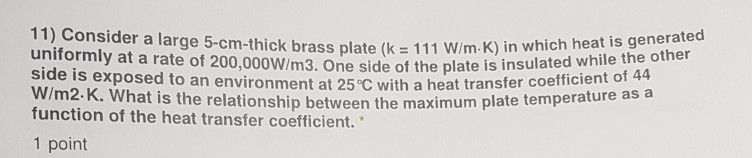11) Consider a large 5-cm-thick brass plate (k111 W/m-K) in whic uniformly at a rate of 200,000W Im3. One side of the plate is insulated while the other exposed to an environment at 25 C with a heat transfer coefficient of What is the relationship between the maximum plate temperature as W/m2.K. function of the heat transfer coefficient." 1 point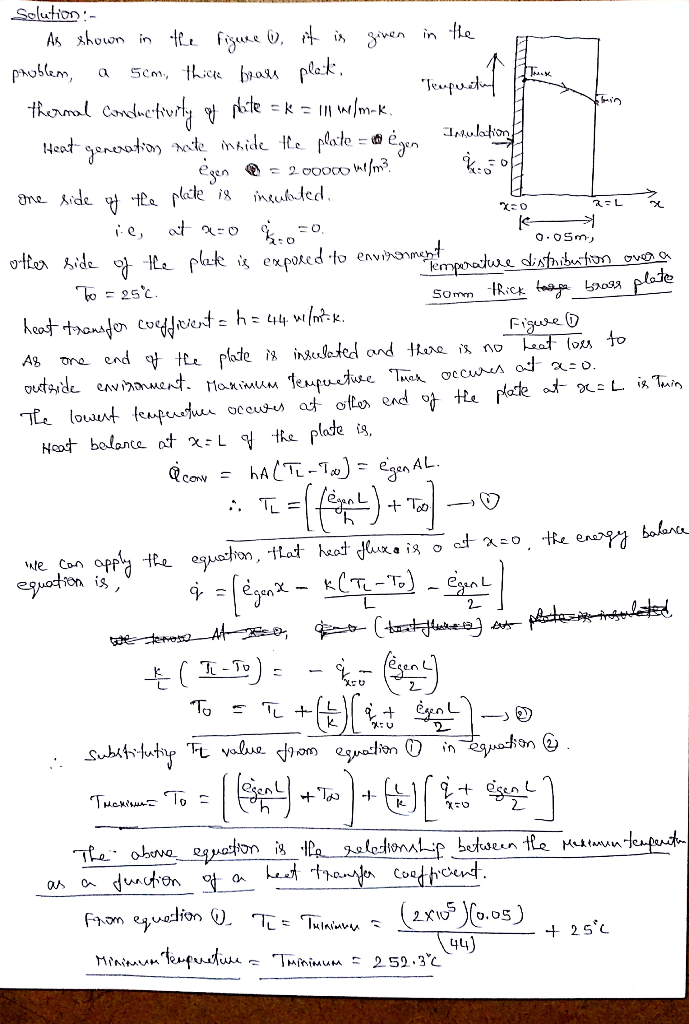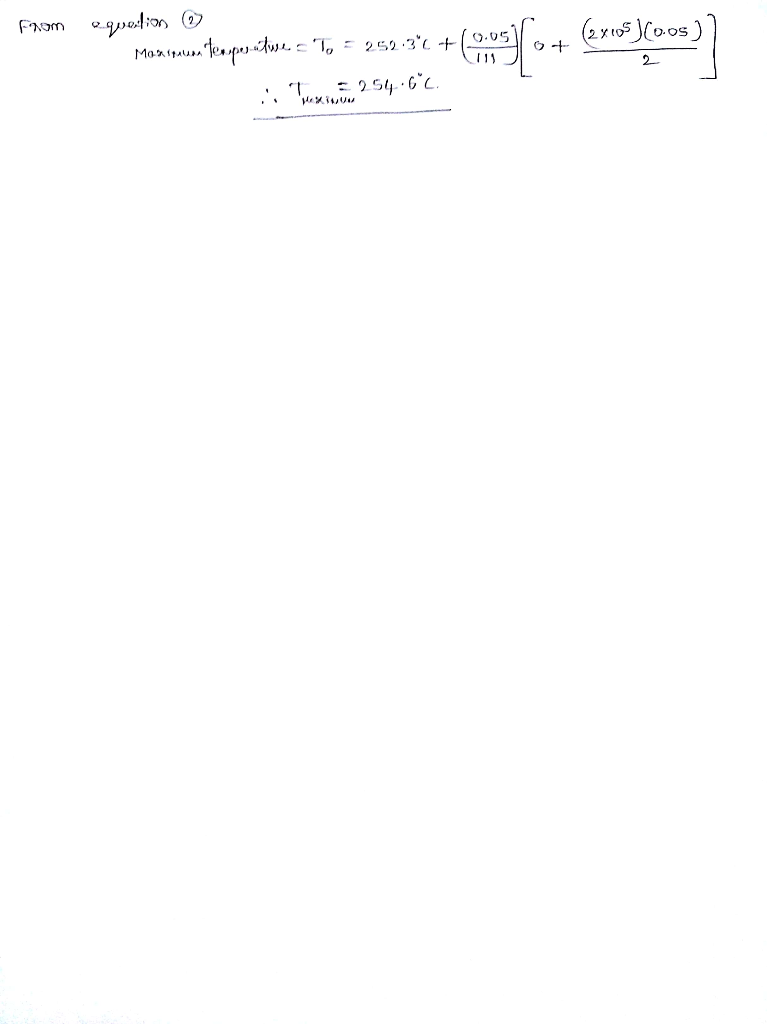##### Add Answer of: 11) Consider a large 5-cm-thick brass plate (k111 W/m-K) in whic uniformly at a rate of 200,000W Im3. One side of th...
Similar Homework Help Questions
• ### A large wall made of 10-cm-thick solid brick (k = 0.8 W/m.K, p 2000 kg/m3, and p 800 J/kg.K) is o...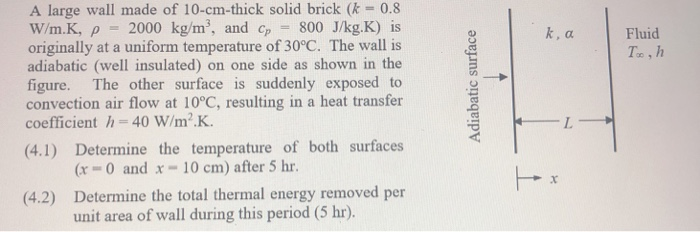Heat transfer question. A large wall made of 10-cm-thick solid brick (k = 0.8 W/m.K, p 2000 kg/m3, and p 800 J/kg.K) is originally at a uniform temperature of 30°C. The wall is adiabatic (well insulated) on one side as shown in the figure. The other surface is suddenly exposed to convection air flow at 10°C, resulting in a heat transfer coefficient h=40W/m2.K. Fluid (4.1) Determine the temperature of both surfaces Gr 0 and x 10 cm) after 5 hr...

• ### Your answer is incorrect. Try again. A square aluminum plate 5 mm thick and 160 mm on a side is h...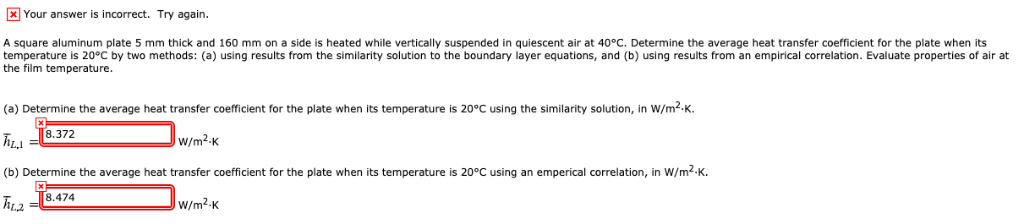Your answer is incorrect. Try again. A square aluminum plate 5 mm thick and 160 mm on a side is heated while vertically suspended in quiescent air at 40°C. Determine the average heat transfer coefficient for the plate when its temperature is 20°C by two methods: (a) using results from the similarity solution to the boundary layer equations, and (b) using results from an empirical correlation. Evaluate properties of air at the film temperature (a) Determine the average heat transfer...

• ### Cold conditioned air at 10°C is flowing inside a 1.5 cm thick square aluminum (k = 240 w/mK) duct...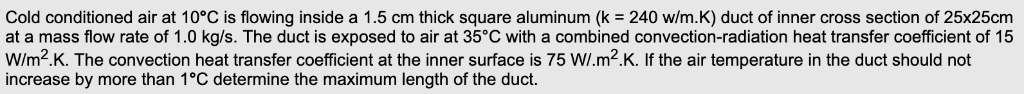Cold conditioned air at 10°C is flowing inside a 1.5 cm thick square aluminum (k = 240 w/mK) duct of inner cross section of 25x25cm at a mass flow rate of 1.0 kg/s. The duct is exposed to air at 35°C with a combined convection-radiation heat transfer coefficient of 15 W/m2K. The convection heat transfer coefficient at the inner surface is 75 W/.m2.K. If the air temperature in the duct should not increase by more than 1°C determine the maximum...

• ### H8-1: An 8-m-internal-diameter spherical tank made of 1.5-cm-thick stainless steel (k-15 W/m K) i...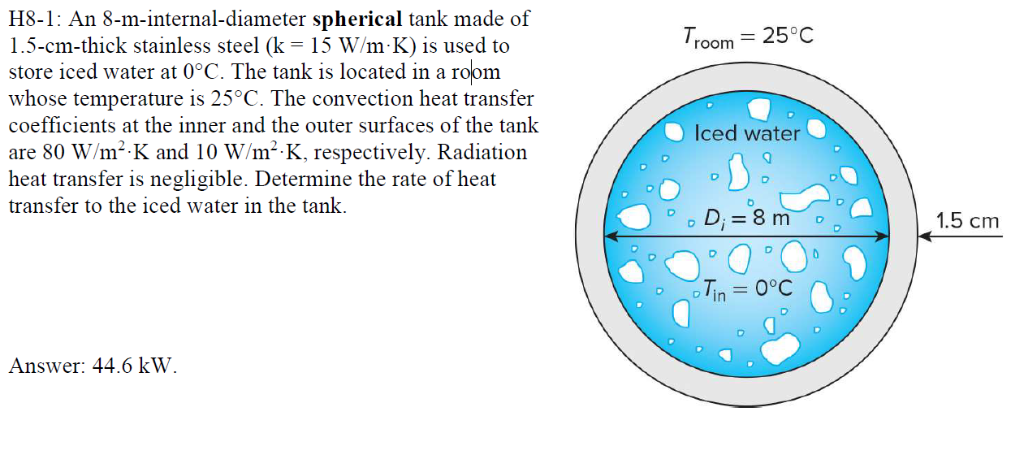H8-1: An 8-m-internal-diameter spherical tank made of 1.5-cm-thick stainless steel (k-15 W/m K) is used to store iced water at 0°C. The tank is located in a room whose temperature is 25°C. The convection heat transfer coefficients at the inner and the outer surfaces of the tank are 80 W/m2 K and 10 W/m2 K, respectively. Radiatior heat transfer is negligible. Determine the rate of heat transfer to the iced water in the tank. room 25°0c T. Iced water 0...

• ### Heat Transfer Problem2A plane wall, 7.5 cm thick, generates heat internally at the rate of w/m^3. One side of the wall isinsulated, and the other side is exposed to an environment at 90 degrees celsius. The convection heat transfer coefficient between the wall and the environment is500w/m^2 k. If the thermal conductivity of the wall is 12 w/m k, calculate the maximum temperature in the wall.

• ### How do I set up this problem? Problem 5 - 24

Consider a large uranium plate of thickness 5 cm and thermal conductivity k = 34W/mK in which heat is generated uniformly at a constant rate of e = 6 X 10^5 W/m^3. Oneside of the plate is insulated while the other side is subjected to convection to an environment at 30C with a heat transfer coefficient of h = 60W/m^2K. Consideringsix equally spaced nodes with a nodal spacing of 1cm, (a) obtain the finite difference formulation of this problem and...

• ### Reviewer Score 3. A plane wall of thickness 0.12m and thermal conductivity 40W/m K having uniform volumetric ene...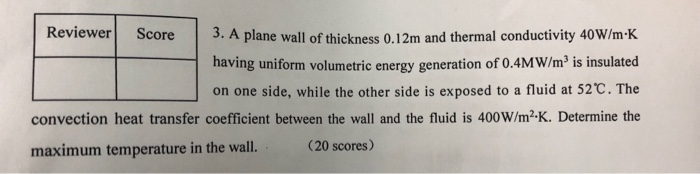Reviewer Score 3. A plane wall of thickness 0.12m and thermal conductivity 40W/m K having uniform volumetric energy generation of 0.4MW/m3 is insulated on one side, while the other side is exposed to a fluid at 52 C. The convection heat transfer coefficient between the wall and the fluid is 400W/m2-K. Determine the (20 scores) maximum temperature in the wall. 4. r,rod OA rotates with uniform o o. At the moment, AB- 6r Signatory Score leration of block B at...

• ### lifesaver

III. An aluminum plate 4 mm thick is mounted in a horizontal position, and its bottom surface is well insulated. A special, thin coating is applied to the top surfacesuch that it absorbed 90% of any incident solar radiation, while having an emissivity of 0.20. The density ? and specific heat C of aluminum are known to be 2,700kg/m3 and 900 J/kg.oK, respectively. Consider conditions for which the plate is at a temperature of 25oC and its top surface is...

• ### cryogenic fluid flowing through SiC plate. Insulation on both sides.

In an experiment a porous plate of silicon carbide (ksiC = 490 W/m-­?K), with a heat transfer area of 0.5 m2, 15­?cm thick is cooled by a cryogenic fluid. Assume thatthe cryogenic fluid’s volume is small and does not effect the material properties of the SiC plate. The cryogenic fluid acts like a heat sink in the plate with somevolumetric heat rate (W/m3). The walls of the plate are insulated, 5-­?cm thick on each side and kin = 0.05 W/m-­?K....

• ### LIFESAVER

III. An aluminum plate 4 mm thick is mounted in a horizontal position, and its bottom surface is well insulated. A special, thin coating is applied to the top surfacesuch that it absorbed 90% of any incident solar radiation, while having an emissivity of 0.20. The density and specific heat C of aluminum are known to be 2,700 kg/m3and 900 J/kg K, respectively. Consider conditions for which the plate is at a temperature of 25oC and its top surface is...

Need Online Homework Help?